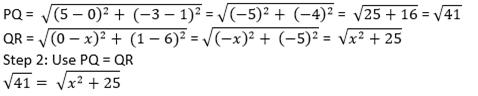• +91 9971497814
• info@interviewmaterial.com

# Coordinate Geometry Ex-7.1 Interview Questions Answers

### Related Subjects

Question 1 : Find the distance between the following pairs of points:

(i) (2, 3), (4, 1)

(ii) (-5, 7), (-1,3)

(iii) (a, b), (- a,– b)

Solution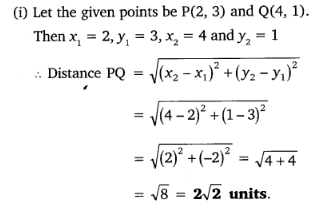Solution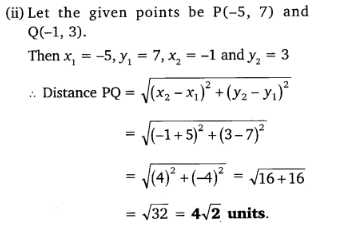Solution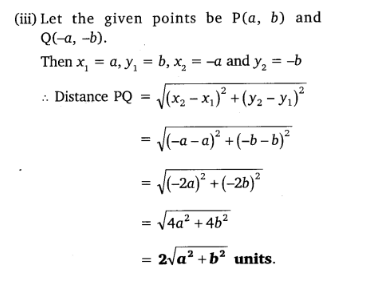Question 2 : Find the distance between the points (0, 0)and (36, 15). Can you now find the distance between the two towns A and Bdiscussed in Section 7.2.

Let us consider, town A at point (0, 0).Therefore, town B will be at point (36, 15).

Distance between points (0, 0) and (36, 15)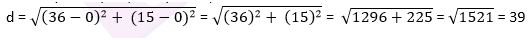The distance between town A and B will be 39km.

Question 3 :

Determine if thepoints (1, 5), (2, 3) and (-2, -11) are collinear.

Answer 3 :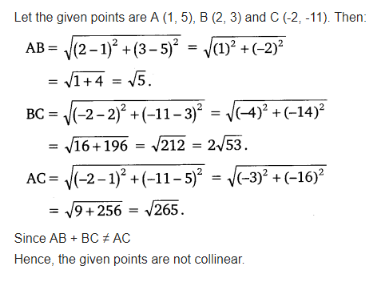Question 4 :

Check whether (5, – 2), (6, 4) and (7, – 2) are the vertices of an isosceles triangle.

Since two sides of any isosceles triangle areequal. To check whether given points are vertices of an isosceles triangle, wewill find the distance between all the points.

Let the points (5, – 2),(6, 4), and (7, – 2) are representing the vertices A, B, and C respectively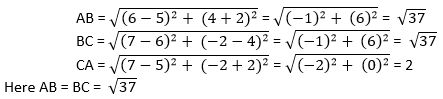This implies, whether given points arevertices of an isosceles triangle.

Question 5 :

In a classroom, 4friends are seated at the points A, B, C and D as shown in Fig. 7.8. Champa andChameli walk into the class and after observing for a few minutes Champa asksChameli, “Don’t you think ABCD is a square?” Chameli disagrees. Using distance formula,find which of them is correct.

Chameli walk into the class and after observing for a few minutes Champa asks Chameli, “Don’t you think ABCD is a square?” Chameli disagrees. Using distance formula, find which of them is correct.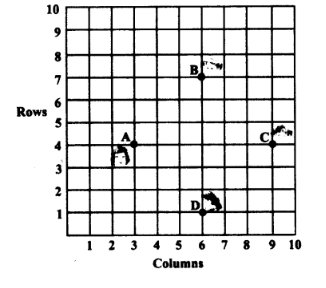Solution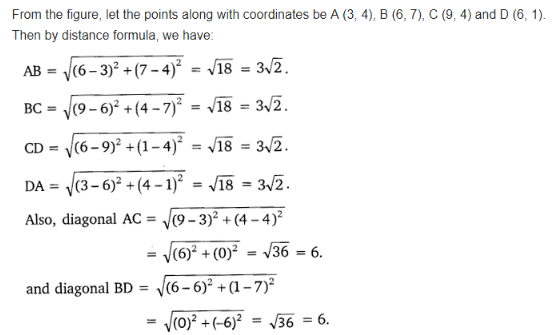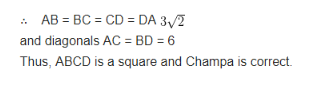Question 6 : Name the type of quadrilateral formed, if any, by the following points, and give reasons for your answer:

(i) (- 1, – 2), (1,0), (- 1, 2), (- 3, 0)

(ii) (- 3, 5), (3,1), (0, 3), (- 1, – 4)

(iii) (4, 5), (7,6), (4, 3), (1, 2)

Solution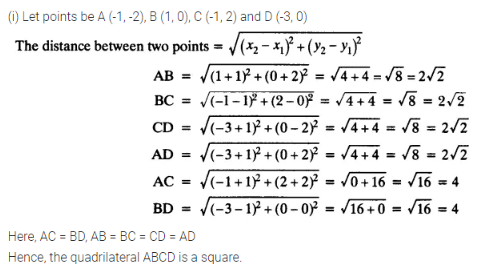Solution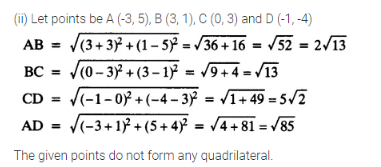Solution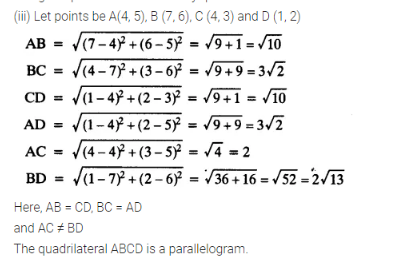Question 7 :

Find the point onthe x-axis which is equidistant from (2, – 5) and (- 2, 9).

Answer 7 :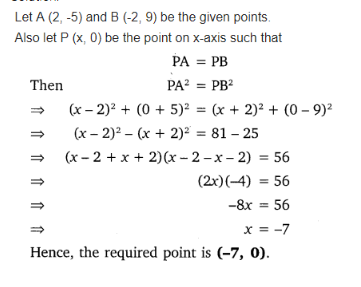Question 8 :

Find the values of yfor which the distance between the points P (2, – 3) and Q (10, y) is 10 units.

Given: Distance between (2, – 3) and (10, y)is 10.

Using distance formula,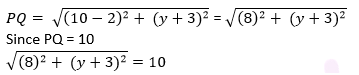Simplify the above equation and find the valueof y.

Squaring both sides,

64 +(y+3)2 = 100

(y+3)= 36

y + 3 = ±6

y + 3 = +6 or y + 3 = −6

Therefore, y = 3 or -9.

Question 9 :

If Q (0, 1) isequidistant from P (5, – 3) and R (x, 6), find the values of x. Also find thedistance QR and PR.